# 逻辑学探幽 Part5

## 没有逻辑 只有heip

Posted by R1NG on October 16, 2021 Viewed Times

# DPLL

## 5.1 子句 (Clauses)

$\widetilde{L} \overset{\text{def}}{=} \begin{cases} \neg L ~~~ \text{if L is positive} \\ ~~~L ~~~ \text{if L has the form} ~ \neg A\end{cases}$

$\widetilde{\widetilde{L}} = L.$

1. 若 $n=0$, 称这样的子句为 (empty), 用 $\square$ 表示.

2. 若 $n=1$, 此时子句恰由一个文字组成, 称这样的子句为 单位 (unit).

3. 若组成子句的文字中 最多有一个为真, 称这样的子句为 Horn 子句.

4. 类似地, 若组成子句的文字全为真, 称该子句也为 正的, 反之若全为假, 则称该子句为 负的.

1. 显然, 任何单位子句都是 Horn 子句.
2. 一个 正的 子句为 Horn 子句, 当且仅当它或为单位子句, 或为空.
3. 任何 负的 子句都是 Horn 子句.

$I(p) = \begin{cases} 0 ~~~ \text{if literal p is positive} \\ 1 ~~~ \text{if literal p is negative}\end{cases}.$

$A = \bigwedge_{i} \bigvee_{j} L_{i,j}$

$A = \bigvee_{i} \bigwedge_{j} L_{i,j}$

## 5.2 向合取范式的转换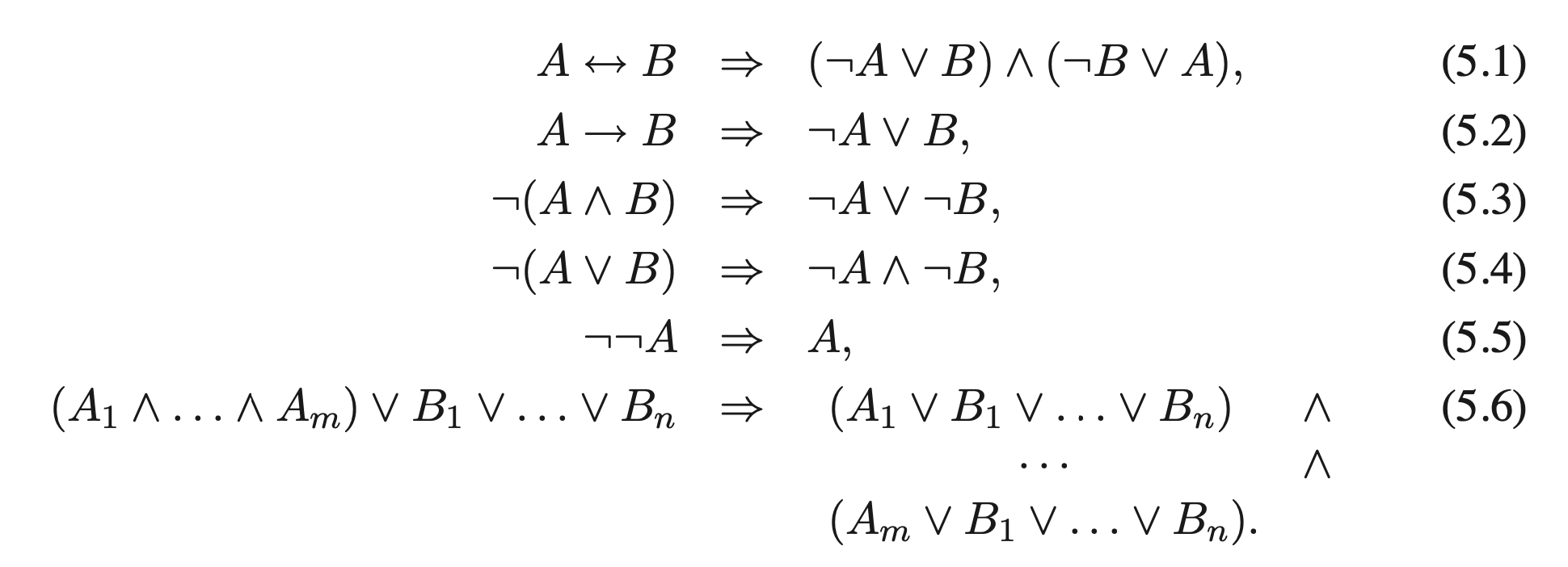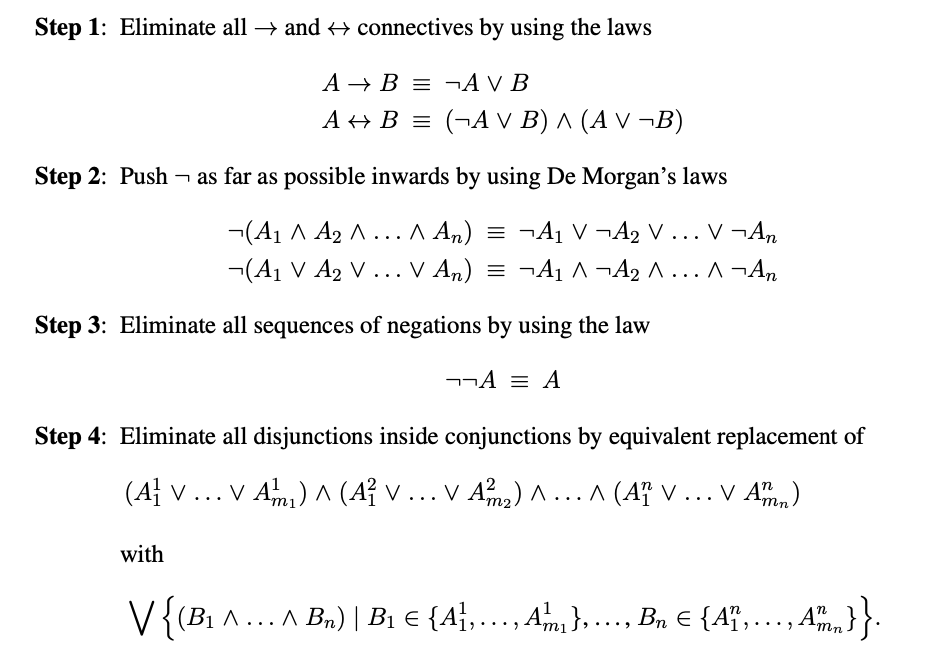## 5.3 子句形式和定义变换

$p_1 \leftrightarrow (p_2 \leftrightarrow (p_3 \leftrightarrow (p_4 \leftrightarrow (p_5 \leftrightarrow p_6))))$

$n \leftrightarrow Q \\ n \leftrightarrow P$

$S' = \{p, n \leftrightarrow p\}$

1. 必要性: 显然可知, $S \cup {n \leftrightarrow B}$ 的任一模型都是 $S$ 的一个模型, 故必要性成立. $\blacksquare$

2. 充分性: 不妨假设 $S$ 在解释 $I$ 下为可满足的. 我们定义新的解释 $I’$ 如下:

$I'(q) \overset{\text{def}}{=} \begin{cases} I(B) ~~~ \text{if} ~q=n \\ I(q) ~~~~ \text{else}\end{cases}$

1. 若 $A$ 本身已经是一系列子句 $C_1, C_2, \cdots, C_n$ 的合取: 则
$S \overset{\text{def}}{=} \{C_1, C_2, \cdots, C_n\}.$
1. 若不然, 对 $A$ 中的每一个不是文字的子式 $B$, 我们定义一个执行转换的函数 $n(B)$:

$n(B) \overset{\text{def}}{=} \begin{cases} B ~~~ \text{if} ~B~ \text{is a literal} \\ p_B ~~~ \text{else}\end{cases}$

并且记 $\widetilde{n}(B)$ 为 $n(B)$ 的否定. 我们基于如下的规则定义 $p_B \leftrightarrow B$:

i. 若 $B$ 形为 $B_1 \wedge B_2 \wedge \cdots \wedge B_n$, 则将对变量 $p_B$ 的定义:

$p_B \leftrightarrow n(B_1) \wedge n(B_2) \cdots \wedge n(B_m)$

加入 $S$ 中, 也就是:

\begin{aligned} &\neg p_B \vee n(B_1), \\ &\cdots \\ &\neg p_B \vee n(B_m), \\ &\widetilde{n}(B_1) \vee \cdots \vee \widetilde{n}(B_m) \vee p_B \end{aligned}

ii. 若 $B$ 形为 $B_1 \wedge B_2 \vee \cdots \vee B_n$, 则将对变量 $p_B$ 的定义:

$p_B \leftrightarrow n(B_1) \vee n(B_2) \cdots \vee n(B_m)$

加入 $S$ 中, 也就是:

\begin{aligned} &p_B \vee \widetilde{n}(B_1), \\ &\cdots \\ &p_B \vee \widetilde{n}(B_m), \\ &n(B_1) \vee \cdots \vee n(B_m) \vee \neg p_B \end{aligned}

iii. 若 $B$ 形为 $B_1 \rightarrow B_2$, 则将对变量 $p_B$ 的定义:

$p_B \leftrightarrow (n(B_1) \rightarrow n(B_2))$

加入 $S$ 中, 也就是:

\begin{aligned} &\neg p_B \vee \widetilde{n}(B_1) \vee \widetilde{n}(B_2), \\ &n(B_1) \vee p_B, \\ &\widetilde{n}(B_2) \vee p_B\end{aligned}

iv. 若 $B$ 形为 $\neg B_1$, 则将对变量 $p_B$ 的定义:

$p_B \leftrightarrow \neg n(B_1)$

加入 $S$ 中, 也就是:

\begin{aligned} &\neg p_B \vee \widetilde{n}(B_1), \\ &n(B_1) \vee p_B \end{aligned}

v. 若 $B$ 形为 $B_1 \leftrightarrow B_2$, 则将对变量 $p_B$ 的定义:

$p_B \leftrightarrow (n(B_1) \leftrightarrow n(B_2))$

加入 $S$ 中, 也就是:

\begin{aligned} &\neg p_B \vee \widetilde{n}(B_1) \vee \widetilde{n}(B_2), \\ &\neg p_B \vee \widetilde{n}(B_2) \vee \widetilde{n}(B_1), \\ &n(B_1) \vee n(B_2) \vee p_B, \\ &\widetilde{n}(B_2) \vee \widetilde{n}(B_2) \vee p_B\end{aligned}

1. 若 $A$ 本身已经是一系列子句 $C_1, C_2, \cdots, C_n$ 的合取: 则
$S \overset{\text{def}}{=} \{C_1, C_2, \cdots, C_n\}.$
1. 若不然, 对 $A$ 中的每一个不是文字的子式 $B$, 我们定义一个执行转换的函数 $n(B)$:

$n(B) \overset{\text{def}}{=} \begin{cases} B ~~~ \text{if} ~B~ \text{is a literal} \\ p_B ~~~ \text{else}\end{cases}$

并且记 $\widetilde{n}(B)$ 为 $n(B)$ 的否定. 我们基于如下的规则定义 $p_B \leftrightarrow B$.

i. 若 $B$ 形为 $B_1 \wedge B_2 \wedge \cdots \wedge B_n$, 则将对变量 $p_B$ 的定义加入 $S$ 中:

$p_B \rightarrow n(B_1) \wedge n(B_2) \cdots \wedge n(B_m)$ $n(B_1) \wedge n(B_2) \cdots \wedge n(B_m) \rightarrow p_B$
$\neg p_B \vee n(B_1),$
$\cdots$
$\neg p_B \vee n(B_m)$
$\widetilde{n}(B_1) \vee \cdots \vee \widetilde{n}(B_m) \vee p_B$

ii. 若 $B$ 形为 $B_1 \wedge B_2 \vee \cdots \vee B_n$, 则将对变量 $p_B$ 的定义加入 $S$ 中:

$p_B \rightarrow n(B_1) \vee n(B_2) \cdots \vee n(B_m)$ $n(B_1) \vee n(B_2) \cdots \vee n(B_m) \rightarrow p_B$
$n(B_1) \vee \cdots \vee n(B_m) \vee \neg p_B$ $p_B \vee \widetilde{n}(B_1),$
$\cdots$
$p_B \vee \widetilde{n}(B_m)$

iii. 若 $B$ 形为 $B_1 \rightarrow B_2$, 则将对变量 $p_B$ 的定义加入 $S$ 中:

$p_B \rightarrow (n(B_1) \rightarrow n(B_2))$ $(n(B_1) \rightarrow n(B_2)) \rightarrow p_B$
$\neg p_B \vee \widetilde{n}(B_1) \vee n(B_2)$ $n(B_1) \vee p_B,$
$\widetilde{n}(B_2) \vee p_B$

iv. 若 $B$ 形为 $\neg B_1$, 则将对变量 $p_B$ 的定义加入 $S$ 中:

$p_B \rightarrow \neg n(B_1)$ $\neg n(B_1)\rightarrow p_B$
$\neg p_B \vee \widetilde{n}(B_1)$ $n(B_1) \vee p_B$

v. 若 $B$ 形为 $B_1 \leftrightarrow B_2$, 则将对变量 $p_B$ 的定义加入 $S$ 中:

$p_B \rightarrow (n(B_1) \leftrightarrow n(B_2))$ $(n(B_1) \leftrightarrow n(B_2)) \rightarrow p_B$
$\neg p_B \vee \widetilde{n}(B_1) \vee n(B_2),$
$\neg p_B \vee \widetilde{n}(B_2) \vee n(B_1)$
$n(B_1) \vee n(B_2) \vee p_B,$
$\widetilde{n}(B_2) \vee \widetilde{n}(B_2) \vee p_B$

## 5.4 SAT 和 k-SAT

SAT 为这样的决策问题: 考虑一个有限的, 由子句组成的集合, 若该集合是可满足的, 则该决策问题的答案为 “是”, 反之为 “否”.

1. $2-\text{SAT}$ 问题可在多项式时间内求解.
2. $k-\text{SAT}$ 在 $k \geqslant 3$ 的情况下为一个 $\text{NP}$-完全问题.

## 5.5 单位子句传播法

1. 从 $S$ 中将任何形如 $L \vee C’$ 的子句移除.
2. 将 $S$ 中任何形如 $\widetilde{L}\vee C’$ 的子句替换为 $C’$.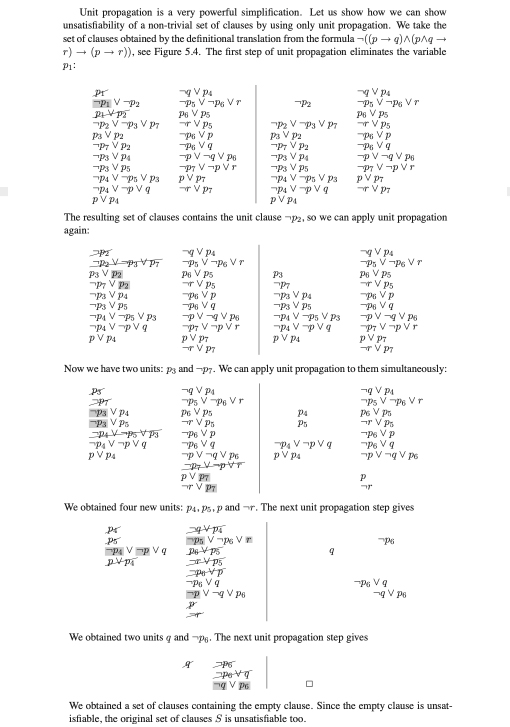## 5.7 DPLL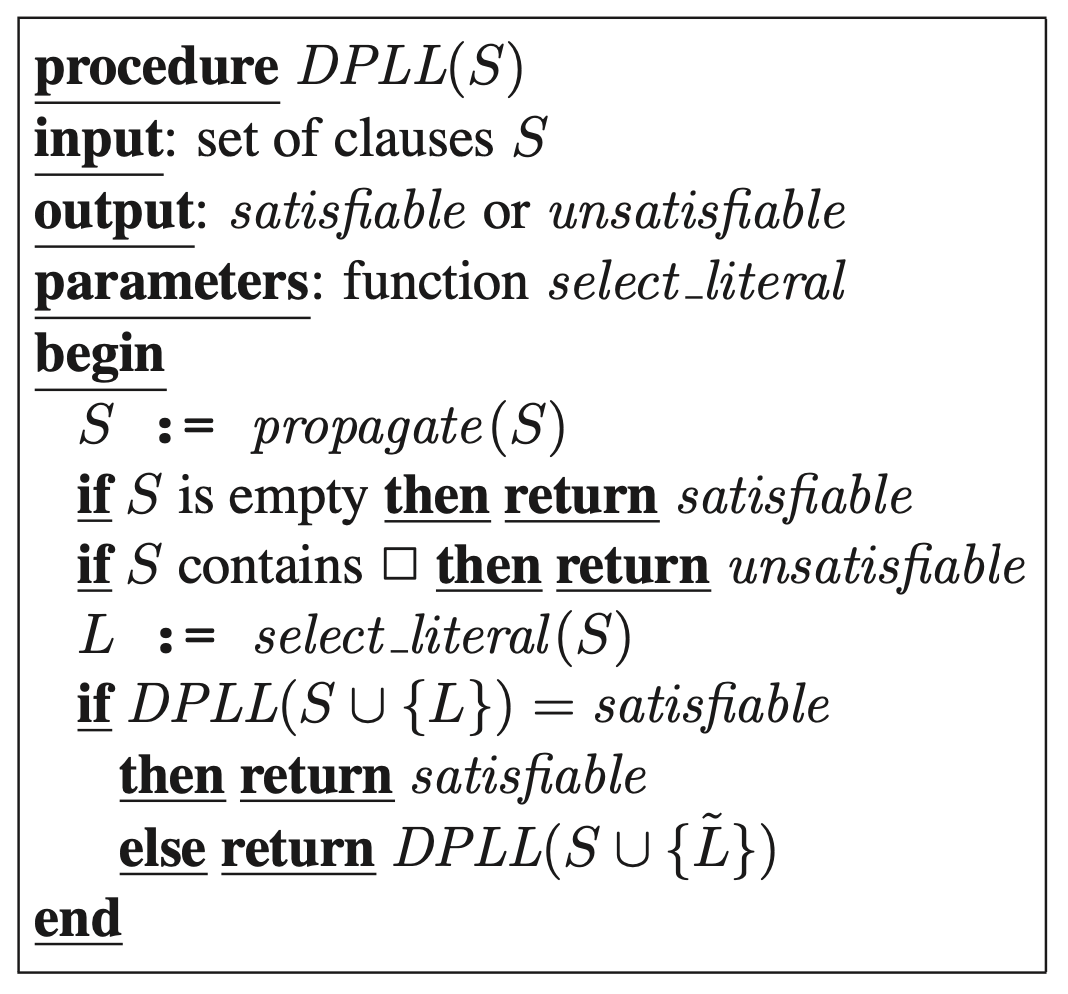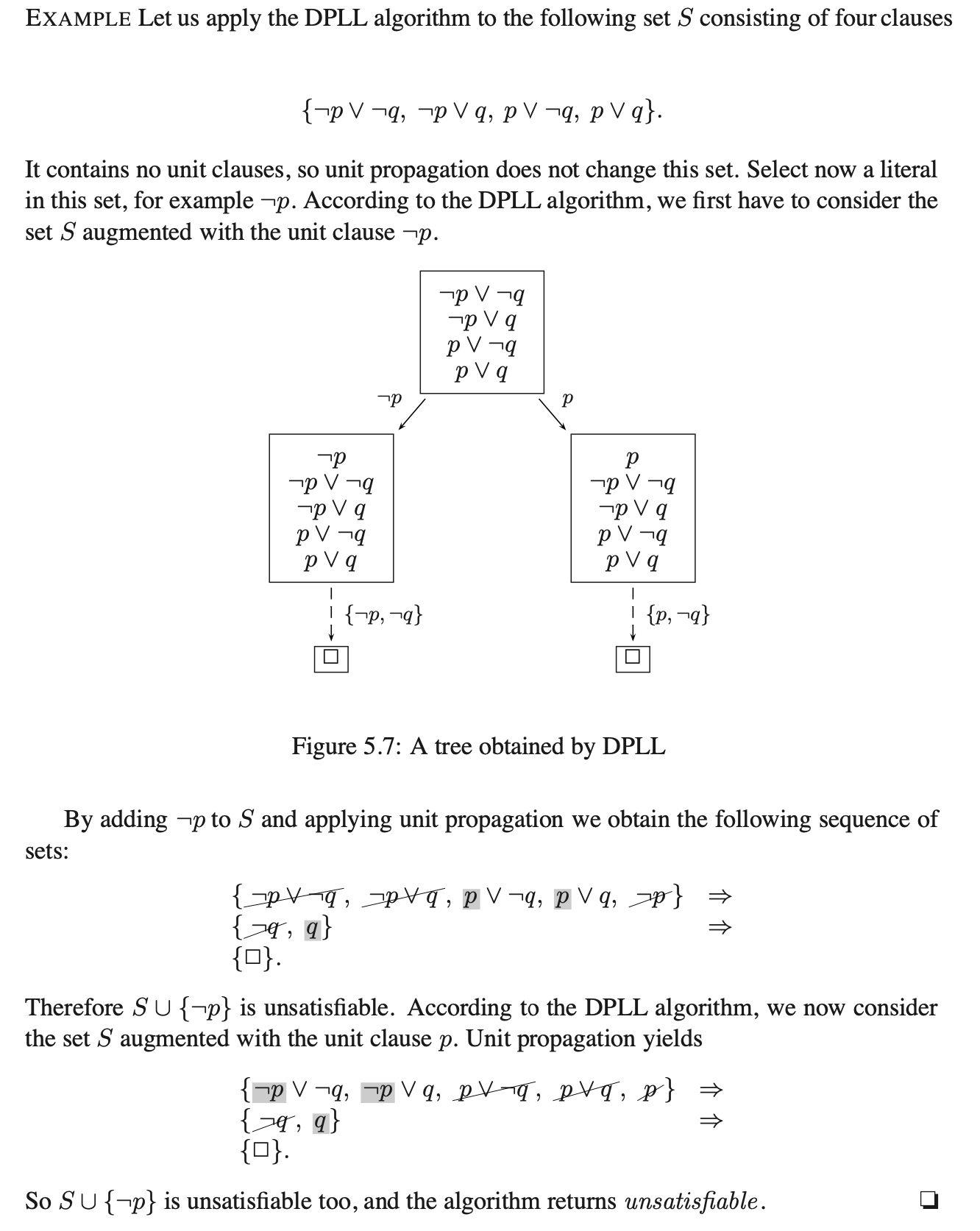DPLL 作用于子句集合 $S$ 得到的结果为 “该集合具备可满足性”, 设 $\mathscr{L}$ 为由所有在 DPLL 的处理决策树中导致最终得到空子句 (空子句集) 的分支上所有文字组成的集合, 则至少存在一个解释 $I$ 使:

$I(p) = \begin{cases} 1~~~ \text{if} ~ p \in \mathscr{L} \\ 0~~~ \text{if} ~ \neg p \in \mathscr{L}\end{cases}.$

## 5.8 优化与实现

DPLL 的核心逻辑已经足够简单以至于难以进一步优化. 行之有效的优化手段一般从算法效率方面入手. 在此我们介绍两种针对 DPLL 逻辑的优化: 重言式消除 (Tautology Elimination) 和纯文本法则 (Pure Literal Rule).

### 纯文本法则

DPLL 中, 我们可以通过计算每个文本 $L$ 在子句集合中出现的次数判断它是否为纯文本.

DPLL 的搜索过程中应用回溯算法会导致它的搜索次数呈指数级别, 因此对 select_literal() 选择下一文本的规则进行优化是至关重要的. 在这里我们不再介绍对算法效率本身的优化方法, 本章到此结束. $\blacksquare$